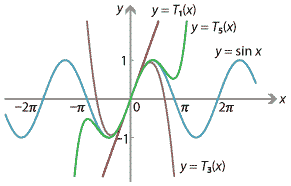### Approximations of functions

We have seen that the equation of the tangent line to the graph $$y=f(x)$$ at the point $$x=a$$ is given by

$y = f(a) + f'(a)\,(x-a).$

Therefore, if we define a linear function $$T_1 (x)$$ as

$T_1(x) = f(a) + f'(a)\,(x-a),$

then $$T_1(x)$$ approximates $$f(x)$$ near $$x=a$$. In fact, since $$T'_1(x) = f'(a)$$, we have

$T_1(a) = f(a) \qquad\text{and}\qquad T'_1(a) = f'(a).$

So the functions $$T_1(x)$$ and $$f(x)$$ agree at $$x=a$$, and their derivatives also agree at $$x=a$$. In this sense, $$T_1(x)$$ is the 'best linear approximation' to $$f(x)$$ at $$x=a$$.

However, there's no reason to stop at linear approximations: we might ask for the best quadratic approximation to $$f(x)$$ at $$x=a$$.

Consider the function

$T_2(x) = f(a) + f'(a)\,(x-a) + \dfrac{f''(a)}{2}\,(x-a)^2.$

This is a quadratic function. Its derivatives are

$T'_2(x) = f'(a) + f''(a)\,(x-a) \qquad\text{and}\qquad T''_2(x) = f''(a),$

so

$T_2(a) = f(a), \qquad T'_2(a) = f'(a) \qquad\text{and}\qquad T''_2(a) = f''(a).$

That is, $$T_2(x)$$ and $$f(x)$$ agree at $$x=a$$, as do their first and second derivatives. In this sense, $$T_2(x)$$ is the best quadratic approximation to $$f(x)$$ at $$x=a$$.

In general, one can check that the function

$T_k (x) = f(a) + f'(a)\,(x-a) + \dfrac{f''(a)}{2!}\,(x-a)^2 + \dfrac{f^{(3)}(a)}{3!}\,(x-a)^3 + \dots + \dfrac{f^{(k)}(a)}{k!}\,(x-a)^k$

is a polynomial of degree $$k$$ which agrees with $$f(x)$$ at $$x=a$$, along with all its first $$k$$ derivatives. That is,

$T_k (a) = f(a), \quad T'_k (a) = f'(a), \quad T''_k (a) = f''(a), \quad \dotsc, \quad T^{(k)}(a) = f^{(k)}(a).$

In this sense, $$T_k (x)$$ is the best polynomial approximation of degree $$k$$ to $$f(x)$$ at $$x=a$$.

The polynomials $$T_k (x)$$, called Taylor polynomials, are very important throughout mathematics and science for approximating functions. Taylor polynomials and their generalisations are studied extensively in university-level mathematics courses.

The following diagram shows some of the first few Taylor polynomials approximating the function $$f(x) = \sin x$$ near $$x=0$$. Note how they provide successively better approximations to $$f(x)$$. (We will see how to differentiate $$\sin x$$ in the module The calculus of trigonometric functions.)Figure : Taylor polynomials approximating $$\sin x$$ at $$x=0$$.

History and applications - The discoverers of calculus